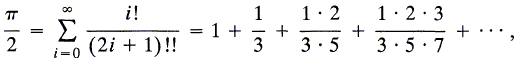## Calculation of the Digits of π by the Spigot Algorithm of Rabinowitz and Wagon

The spigot algorithm for calculating the digits of π and other numbers have been invented by S. Rabinowitz in 1991 and investigate by Rabinowitz and Wagon in 1995. The algorithm generates the digits sequentially, one at a time, and does not use the digits after they are computed. Among other sources, the algorithm is described in a very weill written book by Arndt and Haenel.

The fundamental idea is that of base conversion. In fact the algorithm for conversion between bases outputs one digit at a time as a true spigot algorithm. The great insight was to recognize some of the many known formulas for π as representations of that number in exotic positional system and undertake the task of converting them to the decimal representation.

Both representations are thought in the form utilized by Horner's method. E.g., in the decimal system we have

(1)
 √2 = 1.41421 ... = 1 + 1/10 (4 + 1/10 (1 + 1/10 (4 + 1/10 (2 + 1/10 (1 + 1/10 ( ... )))))), π = 3.14159 ... = 3 + 1/10 (1 + 1/10 (4 + 1/10 (1 + 1/10 (5 + 1/10 (9 + 1/10 ( ... )))))),

But was there a positional system in which π was known? As S. Rabinowitz has realized, there indeed was such a system albeit an unusual one. The starting point was the serieswhich also can be written asor, in the Horner form,This last expression is a representation of π in a system with a mixed-radix base b = (1/3, 2/5, 3/7, 4/9, ...). As the formula shows, in this system the representation of π is exceedingly simple: π = (2; 2, 2, 2, ...)b. However, conversion runs into complications due to the radix not being constant. It may (and does) happen that the algorithm spews as a decimal digit the number 10. In this case, 1 should be carried to the previous digit and, if the latter is 9, even further left. The algorithm accounts for this circumstance.

#### Spigot Algorithm for π

1. Initialize: Let A = (2, 2, 2, 2,... ,2) be an array of length [10n/3] + 1.
2. Repeat n times:
Multiply by 10: Multiply each entry of A by 10.
Put A into regular form: Starting from the right, reduce the ith element of A (corresponding to b-entry (i - 1)/(2i - 1)) modulo 2i - 1, to get a quotient q and a remainder r. Leave r in place and carry q(i - 1) one place left. The last integer carried (from the position where i - 1 = 2) may be as large as 19.
3. Get the next predigit: Reduce the leftmost entry of A (which is at most 109 (= 9 - 10 + 191)) modulo 10. The quotient, q, is the new predigit of π, the remainder staying in place.
4. Adjust the predigits: If q is neither 9 nor 10, release the held predigits as true digits of π and hold q. If q is 9, add q to the queue of held predigits. If q is 10 then:
• set the current predigit to 0 and hold it;
• increase all other held predigits by 1(9 becomes 0);
• release as true digits of π all but the current held predigit.

(Rabinowitz and Wagon argued that it takes [10n/3], where [] is the floor function, digits to calculate n decimal digits. Arndt and Haenel found an inaccuracy in their derivation and changed that to [10n/3] + 1, the value used in the applet.)

### This applet requires Sun's Java VM 2 which your browser may perceive as a popup. Which it is not. If you want to see the applet work, visit Sun's website at https://www.java.com/en/download/index.jsp, download and install Java VM and enjoy the applet.

 What if applet does not run?

(In the applet "Chunk" and "Font size" are output formatting parameters. The digits of π are grouped into chunks for easy reading. The applet enforces a limitation of 50,000 digits - more than a world record some 50 years ago. Elsewhere there is a faster implementation based on another idea of Rabinowitz and Wagon.)

### References

1. S. Rabinowitz, Abstract 863-11-482: A spigot algorithm for π, Abstracts Amer. Math. Society 12 (1991) p. 30.
2. S. Rabinowitz, S. Wagon, A Spigot Algorithm for the Digits of π, The American Mathematical Monthly, Vol. 102, No. 3. (Mar., 1995), pp. 195-203.
3. J. Arndt, C. Haenel, π Unleashed, Springer, 2000• Expansion of Integers in an Integer Base
• Base (Binary, Decimal, etc.) Converter
• Base Converter
• Implementation of Base Conversion Algorithms
• Conversion of Fractions in Various Bases
• Scoring: the simplest of the impartial games
• History of the Binary System
• Addition and Multiplication Tables in Various Bases
•